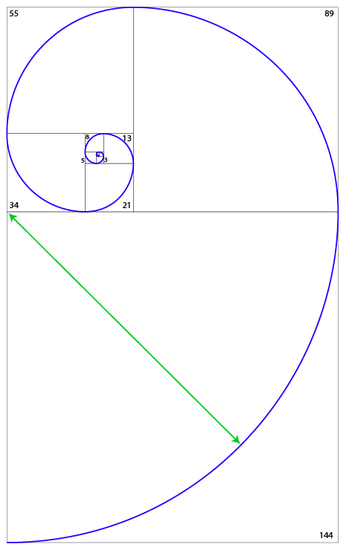﻿ Phi Spiral and Nodes | Cosmometry

# Phi Spiral and Nodes

Phi Spiral

The phi spiral is derived from a very simple process of building an expanding set of adjacent squares of increasing size based upon the Fibonacci sequence, then drawing a quarter-circle arc within each square with a radius equal to the edge length of each respective square (see Illustration 2). Although it always starts at 0 (the singularity center point), it can expand infinitely with ever greater degree of precision approaching the phi ratio with each iteration.Illustration 2: Phi spiral showing arc radius

Nodes

Where the resulting spiral intersects the corner of each new square as it expands is called a node. Each node is a phi-ratio farther out along the spiral from the center than the previous one. Starting from the center as zero, each node is then labeled consecutively as 1, 2, 3, 4, 5, 6… etc (see Illustration 3). Nodes are useful for determining the fractal portion of the phi spiral that is being used by nature in some instances (see Multi-Frequency Phi Spirals below).Illustration 3: 13-node spiral

PREV | NEXT